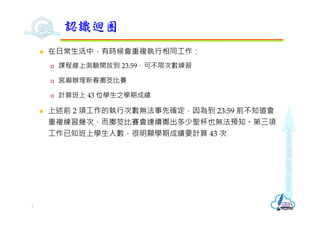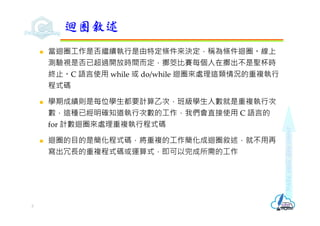Se está descargando tu SlideShare. ×
Anuncio
Anuncio
Anuncio
Anuncio
Anuncio
Anuncio
Anuncio
Anuncio
Anuncio
Anuncio
AnuncioCargando en…3
×

1 de 33 Anuncio

# C語言迴圈作業

Anuncio
Anuncio

### C語言迴圈作業

1. 1. 迴圈作業  迴圈作業  計數迴圈for  前測式迴圈while  後測式迴圈do/while  無窮迴圈  巢狀迴圈  使用continue及break改變迴圈的執 行流程 Revised on July 25, 2021
2. 2. Make each day count  在日常生活中，有時候會重複執行相同工作：  課程線上測驗開放到 23:59，可不限次數練習  宮廟辦理新春擲筊比賽  計算班上 43 位學生之學期成績  上述前 2 項工作的執行次數無法事先確定，因為到 23:59 前不知道會 重複練習幾次，而擲筊比賽會連續擲出多少聖杯也無法預知。第三項 工作已知班上學生人數，很明顯學期成績要計算 43 次 認識迴圈 2
3. 3. Make each day count  當迴圈工作是否繼續執行是由特定條件來決定，稱為條件迴圈。線上 測驗視是否已超過開放時間而定，擲筊比賽每個人在擲出不是聖杯時 終止。C 語言使用 while 或 do/while 迴圈來處理這類情況的重複執行 程式碼  學期成績則是每位學生都要計算⼄次，班級學生人數就是重複執行次 數，這種已經明確知道執行次數的工作，我們會直接使用 C 語言的 for 計數迴圈來處理重複執行程式碼  迴圈的目的是簡化程式碼，將重複的工作簡化成迴圈敘述，就不用再 寫出冗⻑的重複程式碼或運算式，即可以完成所需的工作 迴圈敘述 3
4. 4. Make each day count  for 敘述是⼀種執行特定次數的迴圈，語法如下： for (初始計數值; 條件運算式; 更新計數值){ 重複作業區塊; } int i, total; for(i = 1; i <= 10; i++){ total += i; }  如果重複作業區塊只有單行敘述，則可以省略大括號 for(i = 1; i <= 10; i++) total += i; 計數迴圈for 1/3 4 條件運算式 重複作業區塊 true false 更新計數值 初始計數值 結束
5. 5. Make each day count  計算 1 到 50 偶數和 int i, even_sum=0; for(i = 1; i <= 50; i++){ if (i % 2 == 0) even_sum += i; } printf("1到50偶數和為%dn", even_sum); 計數迴圈for 2/3 5
6. 6. Make each day count  for 迴圏的次數計數也可採用倒數方式  計算 1 到 50 奇數和 int i, odd_sum = 0; for(i = 50; i >= 1; i--){ if (i % 2 != 0) odd_sum += i; } printf("1到50奇數和為%dn", odd_sum); 計數迴圈for 3/3 6
7. 7. Make each day count  在數論中，水仙花數 (Narcissistic number)，也被稱自戀數、自冪數， 用來描述⼀個N位非負整數，其各位數字的N次方和等於該數本身  153是⼀個「水仙花數」，因為153 1 5 3  1634是⼀個「水仙花數」，因為1634 1 6 3 4  列出所有4位數 (1000~9999) 的自戀數 Lab 尋找4位數的自戀數 1/2 7
8. 8. Make each day count  參考程式 int i, j, k, l, n; printf("4位數的自戀數:n"); for(n = 1000; n < 10000; n++){ i = n / 1000; //分解出千位數 j = n / 100 % 10; //分解出百位數 k = n / 10 % 10; //分解出十位數 l = n % 10; //分解出個位數 if (n == i*i*i*i + j*j*j*j + k*k*k*k + l*l*l*l) printf("%-5dn", n); } Lab 尋找4位數的自戀數 2/2 8
9. 9. Make each day count  while 迴圈是在程式區塊的開頭檢查條件，如果條件為 true 才允許進 入迴圈執行，如果⼀直為 true，就持續重複執行迴圈，直到條件 false 為止，語法如下： while(條件運算式){ 重複作業區塊; }  請注意！在重複作業區塊中⼀定要有程式敘述用來更改條件值到達結束條 件，以便結束迴圈的執行，不然，就會造成無窮迴圈，迴圈永遠不會結束 前測式迴圈while 1/2 9 條件運算式 重複作業區塊 true false 結束
10. 10. Make each day count  計算 1 加到多少時的總和會大於等於 255，因為迴圈執行次數需視運 算結果而定，迴圈執行次數未定，我們可以使用 while 條件迴圈來執 行加總計算 int i = 0, sum = 0; while(sum < 255){ i++; sum += i; } printf("1加到%d時的總和會大於等於255n", --i); 前測式迴圈while 2/2 10
11. 11. Make each day count  歐幾里得演算法 (Euclidean Algorithm)，也稱為輾轉相除法，用來計 算兩個整數的最大公因數 (GCD，Greatest Common Divisor)  gcd 𝑚, 𝑛 gcd 𝑛, 𝑚 , 𝑚 𝑛 𝑚, 𝑛 0 gcd 𝑛, 𝑚%𝑛 , 𝑒𝑙𝑠𝑒  GCD Greatest Common Divisor (34, 10) = 2 Lab 計算GCD與LCM 1/4 11 3 34 10 2 30 8 2 4 2 4 0 3 34 10 30 4 3 34 10 2 30 8 4 2 被除數 除數 被除數 除數 除數 被除數 最大公因數
12. 12. Make each day count  最小公倍數 𝐿𝐶𝑀 𝑛 𝑚 𝐺𝐶𝐷 ⁄  使用 Dev C++ 建立 C 程式專案  輸入二整數，自動計算並顯示 GCD 及 LCM Lab 計算GCD與LCM 2/4 12
13. 13. Make each day count int x, y, r; int a, b, lcm; printf("輸入二整數(空白分隔)："); scanf("%d %d", &x, &y); a = x; b = y; while (y != 0) { r = x % y; x = y; y = r; } printf("%d與%d的最大公因數為%dn", a, b, x); printf("%d與%d的最小公倍數為%dn", a, b, a * b / x); Lab 計算GCD與LCM 4/4 14
14. 14. Make each day count  do/while 迴圈類似 while 迴圈也是⼀種條件迴圈，只是 while 括號的 條件是在迴圈程式區塊的最後，語法如下： do { 重複作業區塊; } while(條件運算式);  注意！因為是程式敘述，所以在 while(條件運算式)括號後需加上「;」分 號 後測式迴圈do/while 1/3 15 條件運算式 重複作業區塊 true false 結束 必須加分號
15. 15. Make each day count  do/while 和 while 條件迴圈的主要差異在迴圈條件檢查時機：  while 迴圈在程式區塊開頭先檢查條件，條件為 true 時，才執行之後程式 區塊的程式碼，重複執行直到條件為 false 為止  有可能 while 迴圏的重複作業區塊部份⼀次也不會被執行  do/while 迴圈會先執行程式區塊的程式碼後才在程式區塊後測試條件，執 行次數是持續執行直到條件為 false 為止  do/while 迴圏的重複作業區塊部份⾄少會被執行⼀次 後測式迴圈do/while 2/3 16
16. 16. Make each day count #include <stdio.h> void test_dowhile(void){ int sum = 0, i; printf("計算輸入之整數總和直到輸入0n"); do{ scanf("%d", &i); sum = sum + i; } while(i != 0); printf("sum = %d", sum); } 後測式迴圈do/while 3/3 17
17. 17. Make each day count  for 迴圈與 while 迴圈都是先檢查條件再決定是否執行迴圏程式碼，因 此彼此可以改寫  計算10!，使用for迴圏 int n, factorial = 1; for(n = 1; n <= 10; n++){ factorial *= n; } printf("10!值為%dn", factorial);  計算10!，使用 while 迴圏 int n, factorial = 1; n = 1; while(n <= 10){ factorial *= n; n++; } printf("10!值為%dn", factorial); 改寫迴圈 1/2 18
18. 18. Make each day count  修改原則  在進入 while 迴圈之前需要自行指定計數器變數的初值  在迴圈程式區塊需要自行使用程式碼增減計數器變數值 改寫迴圈 2/2 19 設定初值 迴圈條件 更新計數
19. 19. Make each day count  無窮迴圈 (endless loops) 是指迴圈不會結束，它會無止境的⼀直重複 執行迴圈的程式區塊  for 無窮迴圈 for( ; ; ){ ... }  while 無窮迴圈 while(1){ ... } 無窮迴圈 1/2 20
20. 20. Make each day count  當計數器變數或條件出了問題，也會造成無窮迴圈，這種非預期的狀 況就要費心檢查修正程式 int i = 1, sum = 0; while(i <= 50){ sum += i; i + 2; } 無窮迴圈 2/2 21
21. 21. Make each day count  迴圈中也可以用另⼀個迴圈處理工作，這樣的迴圈也被稱為巢狀迴圈。 C 語言的巢狀迴圈可以有二或二層以上 for(i = 1; i <= 3; i++){ //for外層迴圈 for(j =1 ; j <=5 ; j++){ //for內層迴圈 printf("i=%d j=%dn", i, j); } }  上述迴圈共有兩層，第⼀層 for 迴圈執行 3 次，第二層 for 迴圈是執行 5 次，兩層迴圈共執行 15 次 巢狀迴圈 22 第一層迴圈的i值 第二層迴圈的j值 離開迴圈的i值 1 1 2 3 4 5 1 2 1 2 3 4 5 2 3 1 2 3 4 5 3
22. 22. Make each day count  九九乘法表 Lab 輸出九九乘法表 1/2 23
23. 23. Make each day count  參考程式 int i, j; for(i = 2; i <= 9; i++){ for(j = 1; j <= 9; j++){ printf("%2d *%2d = %2dt", i, j, i * j); if(j % 3 == 0) printf("n"); } printf("n"); } Lab 輸出九九乘法表 2/2 24
24. 24. Make each day count  ㄧ個數如果恰巧等於他的因數之和，稱為「完美數」  6 = 1 + 2 + 3  28 = 1 + 2 + 4 + 7 + 14 Lab 尋找完美數 1/2 25
25. 25. Make each day count  參考程式 int n, i, sum; int mn, mx; printf("輸入起始值："); scanf("%d", &mn); printf("輸入結束值："); scanf("%d", &mx); printf("從%d到%d之間的完美數有：", mn, mx); for(n = mn; n <= mx; n++){ for(i = 1, sum = 0; i <= n / 2; i++) if(n % i == 0) sum = sum + i; if(sum == n) printf("%d ", n); } Lab 尋找完美數 2/2 26
26. 26. Make each day count  使用巢狀迴圈印出以下三角形圖案  第 n 層印出「2 × n - 1」個 * 符號  第 n 層，在*前面空出「圖案高度 - n」個空格 Lab 列印三角形圖案 1/2 27 * *** ***** ******* ********* *********** *************
27. 27. Make each day count  參考程式 int height=7; int i,j; for(i = 0; i < height; i++){ for(j = height - i; j > 0; j--) //左半三角形 printf(" "); for(j = 0; j < (i * 2 + 1); j++) //三角形主體 printf("*"); //右半三角形不需處理 printf("n"); } Lab 列印三角形圖案 2/2 28 三 角 形 主 體 左 半 三 角 形 右 半 三 角 形 * * * * * * * * * * * * * * * * * * * * * * * * * * * * * * * * * * * * * * * * * * * * * * * * *
28. 28. Make each day count  continue 敘述可以⾺上繼續執行下⼀次迴圈，而不執行程式區塊中位 在 continue 敘述之後的程式碼 使用continue敘述繼續迴圈 1/2 29 1 2 3 4 5 6 while(boolean-expression){ statement; ... if(boolean-expression)continue; statement; } 直接執行下一個while判斷
29. 29. Make each day count  列出 1 到 10 之間的偶數 printf("1到10之間的偶數："); for(i = 1; i <= 10; i++){ if((i % 2) == 1) continue; printf("%dt", i); }  計算 1 到 50 中，不是 5 的倍數之數字總合 int i, sum = 0; for(i = 1; i <= 50; i++){ if(i % 5 == 0) continue; sum += i; } printf("1到50，非5的倍數和為%dn", sum); 使用continue敘述繼續迴圈 2/2 30
30. 30. Make each day count  break 敘述會強迫終止 for、while 和 do/while 迴圈的執行  雖然迴圈敘述都會設計結束條件，但是，有時我們需要在特定情況下 終止迴圏，此時就可以使用 break 敘述並搭配 if 條件敘述來實現 使用break敘述終止迴圈 31 1 2 3 4 5 6 7 for(init-expression; cond-expression; loop-expression){ statement; if(boolean-expression) break; statement; ... } statement; 強制脫離for迴圏
31. 31. Make each day count  質數：大於1的自然数中，除了1和該数自身外，無法被其他自然数整 除的数 int i, n; printf("請輸入數字："); scanf("%d", &n); for(i = 2; i < n; i++){ if(n % i == 0) break; } if(i < n) printf("%d可被%d整除，不是質數n", n, i); else printf("%d是質數n", n); Lab 檢驗質數 32
32. 32. Make each day count  和數⼀定是⾄少兩個數相乘 (⼀小⼀大)，兩個數越來越接近，在完全 平方數時會相等，所以只要檢查根號以下是否存在因數就可以了 int i, n; printf("請輸入數字："); scanf("%d", &n); for(i = 2;i <= sqrt(n); i++){ if(n % i == 0) break; } if(i <= sqrt(n)) printf("%d可被%d整除，不是質數n", n, i); else printf("%d是質數n", n); Lab 檢驗質數 (進階版) 33
33. 33. Make each day count  使用Dev C++建立C程式專案，設計程式完成以下功能  專案名稱：學號_6  假設有⼀隻蝸⽜白天會往上爬，晚上會稍微下滑  由鍵盤輸入樹的高度 (到小數點1位)，白天蝸⽜上爬的距離 (到小數點1位) 及晚上蝸⽜下滑的距離 (到小數點1位)  設計程式顯示蝸⽜爬到樹頂的過程 實作練習蝸牛爬樹 34